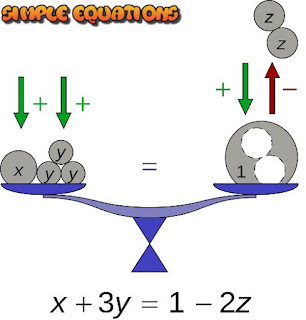## Simple Equations

Worksheet based on NCERT Chapter on Simple Equations

Fill in the blanks

The equation 2x - 5 - 20 is an example of ___________ equation.

The degree of an unknown means the _________ of the unknown.

(a + b)2 = a2 + b2 + 2ab is an example of ________.

A quantity which has a fixed numerical value is called _________.

If RHS and LHS expressions are interchanged will the equation remain ______ (same/different).

While solving an equation if we add a quantity to one side, we must ________ the same quantity to the other side.

If p + 3 = 9 then value of p is ________

The difference between two consecutive odd numbers is _________

_________ values means one after the other in regular order.

An __________ is a condition of equality between two mathematical expressions.

if q ÷ 6 = 7, the value of q is _________.

The sum of two numbers is 33. If one number is 18, what is the other number?

The value of the variable for which the equation is satisfied is called the ______ of the equation.

Form an equation if on adding 3 to a number the result is 4.

Find the measure of each angle of an equilateral triangle.

1: linear
2: power
3: identity
4: constant
5: same
7: 6
8: 2
9: consecutive
10: equation
11: 42
12: 15
13: solution
14: p + 3 = 4
15: 60°1.2.3.